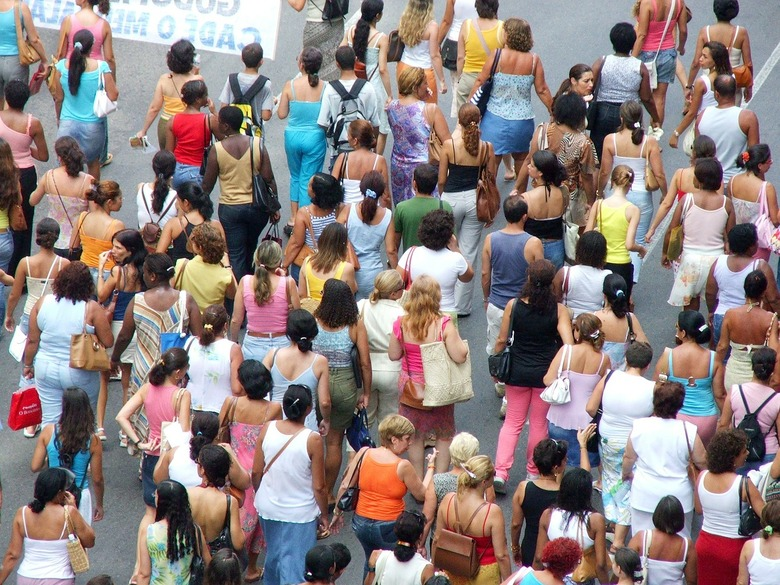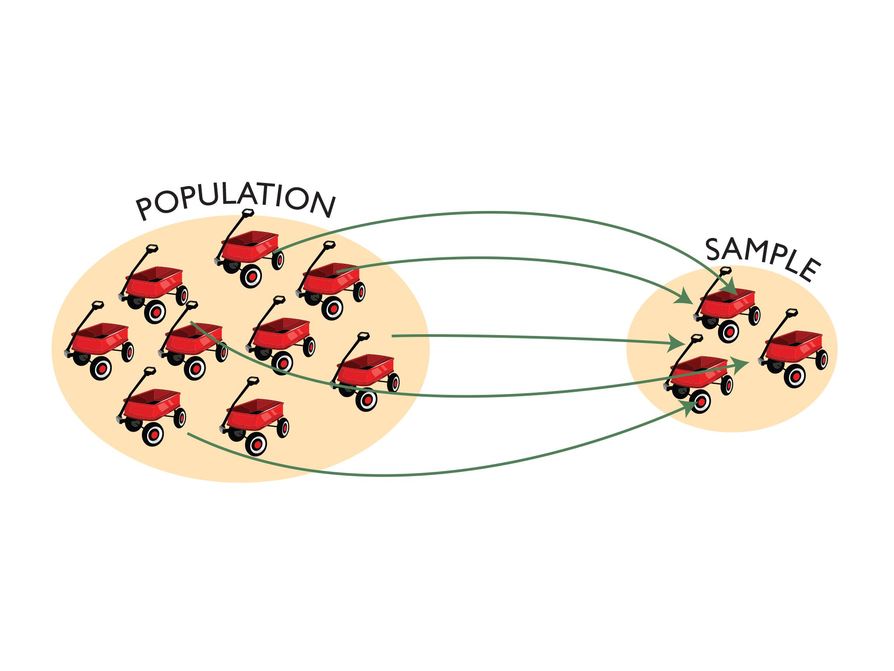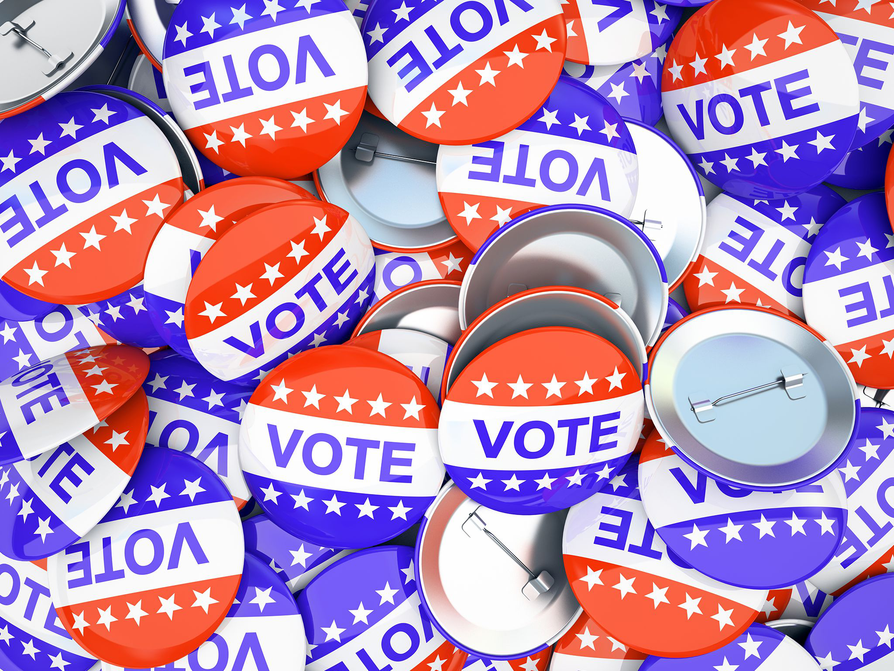Subject:
Statistics and Probability
Material Type:
Lesson Plan
Level:
Middle School
7
Provider:
Pearson
Tags:
Language:
English
Media Formats:
Text/HTML

# Sampling In Relation To Probability## Overview

Students are introduced to the concept of sampling as a method of determining characteristics of a population. They consider how a sample can be random or biased, and think of methods for randomly sampling a population to ensure that it is representative.

The idea of sampling is connected to probability; a relatively small set of data (a random sample/number of trials) can be used to generalize about a population (or determine probability). A larger sample (more trials) will give more confidence in the conclusions, but how large of a sample is needed?

Students also discuss what random means and how to generate a random sample. Random samples are compared to biased samples and give insight into how statistics can be misleading (intentionally or otherwise).

# Key Concepts

Random samples are related to probability. In probability, the number of trials is a sample used to generalize about the probability of an event. The results in probability are random if we are looking at equally likely outcomes. If a data sample is not random, the conclusions about the population will not reflect it.

Terminology introduced in this lesson:

• population: the entire set of objects that can be considered when asking a statistical question
• sample: a subset of a population; can be random, where each object in the population is equally likely to be in the sample, or biased, where not every object in the population is equally likely to be in the sample

# Goals and Learning Objectives

• Introduce sampling as a method to generalize about a population.
• Discuss the concept of a random sample versus a biased sample.
• Determine methods to generate random samples.
• Understand that biased samples are sometimes used to mislead.

SWD: Some students with disabilities will benefit from a preview of the goals in this lesson. Students can highlight the critical features and/or concepts and will help them to pay close attention to salient information.

# Lesson Guide

Have students discuss the Opening question with a partner, and then have a few share their proposed methods.

# Mathematics

Discuss the Opening question, eliciting from students that since it is not possible to calculate a theoretical probability for this question, it is necessary to survey people and calculate the experimental probability.

Some questions to ask to generate discussion:

• Is it possible to ask everyone in the United States this question?
• If we can only ask a few people, how many is enough?
• How could we select the people to survey?
• What if all of the people surveyed were from one town?
• What if most of the people surveyed were women/men?
• What if most of the people surveyed were over/under 21?

ELL: Be aware that all students may be unfamiliar with where the Bahamas are located. It is important to provide culturally relevant examples in order for students to understand and connect with the mathematics. Make sure students can see where the Bahamas are located.

# Vacation in the Bahamas

Estimate the probability that a person from the United States chosen at random will vacation in the Bahamas this summer.

• How would you go about finding this probability?# Lesson Guide

Explain that taking a sample from a population is similar to conducting a probability experiment. We know when conducting an experiment that after a certain number of trials the probability is pretty well known and more trials are not needed. Similarly, when the entire population does not need to be surveyed, it is necessary to select a large enough sample to draw a reasonable conclusion.

Introduce the term random sample, a subset of a population that is a randomly chosen and representative of the population. If the sample does not represent the population, it a biased sample. Draw parallels to probability and the idea of randomly chosen numbers in the Number Cube interactive. If each number on the number cube was not equally likely to be chosen, then the results would not have represented the theoretical probability.

Have students brainstorm a few methods for choosing a sample randomly:

• Rolling a number cube
• Flipping a coin
• Spinning a numbered spinner
• Pulling pieces of paper out of a hat

ELL: During class discussions, make sure you provide wait time (5–10 seconds) and acknowledge student responses, both verbally and with gestures. In addition, provide guiding questions in writing as a reference for ELLs.

SWD: Consider the prerequisite skills for this lesson. Students with disabilities may need direct instruction or guided practice with the skills needed to complete this lesson. Make sure that students understand vocabulary used in the activities in this lesson. Students should understand these domain-specific term/definitions:

Pre-teach these terms to students with disabilities.

• population
• sample
• random
• biased

# Samples and Populations

Discuss the following with your classmates.

• A sample is part of a larger group of objects or people. The larger group is called a population.
• A population can be any group that is appropriate for a particular situation. For example, a population might be defined as the 5,500 residents of a certain city, or the whole population of the United States. In a factory, the population might be the number of items produced in any one day, or the number of items produced in a year.
• A sample must be selected randomly from the population so as to ensure that there is no bias toward one sector of the population or toward any particular result. In a random sample, every member of a population is equally likely to be selected.# Lesson Guide

Discuss the Math Mission. Students will identify samples and populations, and understand how to choose a random sample from a population.

## Opening

Identify samples and populations, and understand how to choose a random sample from a population.

# Lesson Guide

Students will work with a partner.

ELL: Have students share their observations with each other by working cooperatively. This interaction helps students develop English naturally by interacting with others and explaining their thinking about what makes a good sample (unbiased, random, large enough).

# Mathematical Practices

Mathematical Practice 4: Model with mathematics.

Surveys and sampling are natural connections between mathematics and real-world situations. When considering these types of problems, students must account for both the mathematical requirements (e.g., sample size necessary to give accurate results) and practical needs (e.g., whether or not it is possible to survey the entire population).

# Interventions

Student has trouble getting started.

• What do you know that will help you with this problem?

Student has difficulty identifying the target population.

• What types of items or people are in the sample?
• How could you describe all of those types of items or people?
• Does the sample contain the entire population?

• All the residents of voting age in that city
• All students in the seventh grade class
• All the fish in the lake
• All the lightbulbs produced by the factory in that day.

# Sample of Population

Sample size and population size go together. If the population is not too large, the sample size could be the whole population.

What population might these samples have been taken from?

• Two thousand residents of voting age in a particular city are surveyed about their voting intentions.
• Students in a seventh grade class are surveyed about their favorite music group.
• Scientists catch and tag 10 fish in a lake.
• One hundred of the lightbulbs produced in a factory in one day are surveyed for flaws.# Lesson Guide

Students will work with a partner.

# Mathematical Practices

Mathematical Practice 4: Model with mathematics.

Surveys and sampling are natural connections between mathematics and real-world situations. When considering these types of problems, students must account for both the mathematical requirements (e.g., sample size necessary to give accurate results) and practical needs (e.g., whether or not it is possible to survey the entire population).

• It would be better to survey the population.
• It would be better to survey a sample.
• If there are not too many students in the class, it would be better to survey the population.

# Population or Sample

For each situation, decide whether it would be better to survey the population or a sample.

• The shoe sizes of 12 students
• The ages of people who watch professional basketball on television
• The scores that students in your class receive on a math test

# Lesson Guide

Students will work with a partner to complete the Work Time problems.

# Mathematics

As students work through the problems, prompt them to think about what makes a good sample (unbiased, random, large enough). Also prompt them to think about samples, surveys, and television commercials where data was biased (e.g., four out of five dentists surveyed prefer Brand X toothpaste). There is likely to be a lively discussion as students make the connection to advertising and misleading/biased data.

There are also practical issues when considering sample size. For example, it is more practical to survey your family about their favorite movie than to survey the entire town. In the first instance, the sample can be the entire population, while in the second it probably cannot.

# Mathematical Practices

Mathematical Practice 6: Attend to precision.

Students must have a good understanding of what makes a sample random and what makes a sample biased. They must be able to convey their reasoning from a mathematical perspective.

# Interventions

Student cannot understand why a sample is biased.

• How do you know if a sampling method is random?
• In the sampling method, is every person in the population equally likely to be chosen? Does a random sample and a biased sample have the same probability?

Student cannot come up with a method for random sampling.

• What experiments have you done in the unit so far where all the outcomes are equally likely?

• The target population is everyone who buys Juicy Orange Juice.
• The population is large and spread out over several states, so it would be difficult to find and survey the entire population.
• This will result in a biased sample, because only people who have the time and inclination to call will be surveyed. That would not be a random sample.
• Answers will vary. Possible answer: In each state, select random supermarkets. In each supermarket, select random customers buying orange juice to survey.

# Juicy Orange Juice

The makers of Juicy Orange Juice are proud their juice is sold in supermarkets across ten states.

They want to conduct a survey to determine customer satisfaction with their product.

• Describe the target population of the survey.
• It is more practical for the Juicy Orange Juice Company to survey a sample rather than the population? Explain why.

A company executive suggests printing an invitation on the bottle label asking people to phone the Juicy Orange Juice Company with their opinions of the product.

• Describe what is wrong with the sampling method.
• How would you recommend they determine the sample for the survey?
• Would the population be the whole United States?
• How many people do you estimate would be in the population? Would it make sense to survey all of them?
• What type of people might phone in? Would they be different from other members of the population?# Lesson Guide

Presentations should clearly identify the differences between a sample and a population, as well as offer a method for random sampling.

# Challenge Problem

• Answers will vary. Possible answer: Dial random phone numbers and survey people who are registered to vote and plan to vote in the next presidential election. (This is actually how most election pollsters conduct their polls.)

# Prepare a Presentation

Explain the difference between a sample and a population, and how to choose a random sample from a population. Use your work to illustrate your explanations.

# Challenge Problem

• How would you choose a sample to predict which candidate people will vote for in the next presidential election?

# Lesson Guide

Have student share their answers and justify them.

# Mathematics

Consider these questions for discussion:

• How does the size of the population affect the size of the sample needed?
• What methods could you use to get a random sample?
• How could your method be related to probability?
• Can you think of examples of biased sampling from your own experience?

SWD: During student presentations, provide some students with a note-taking organizer that includes a space for the presenters' answer to the problem, the presenters' explanation of the mathematics, etc. This will help students when they reflect on the presentation during the Ways of Thinking discussion.

# Ways of Thinking: Make Connections

Take notes about students' explanations for samples and populations.

• How does the size of the population affect the size of the sample needed?
• What makes a sample biased?
• What method did you propose to the Juicy Orange Juice Company? Why is your method a good one?
• What types of factors do you need to consider when selecting a random sample?
• Can you think of another example of a population and a random sample for that population?

# Lesson Guide

Have students discuss the summary with a partner before turning to a whole class discussion. Prompt students to give examples of random and biased samples. Use this opportunity to correct or clarify misconceptions.

# Summary of the Math: Sampling Methods

Samples that are not chosen using a random method are likely to be biased toward a certain outcome because they do not represent the population. Here are some examples of non-random sampling:

• Convenience sampling: People who are readily available are chosen, such as people entering a shopping mall being asked if they like shopping
• Self-selected sampling: People are asked to call in or fill out a questionnaire to give their opinion; people who respond are likely to have strong opinions
• Systematic sampling: People are selected according to a system, such as choosing every third person on a list; each person is not equally likely to be chosen

Can you:

• Define sample and population?
• Explain what makes a good sample?